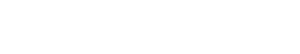# Univariate Analysis

Univariate analysis is used to understand each piece of data within a dataset on its own. This means that each variable is analyzed separately, without considering any other variables in the dataset. Univariate analysis is used to understand the distribution of data, look for patterns, and spot outliers.

It is the simplest form of data analysis, and it is often the first step in data analysis. Sometimes it is used interchangeably with exploratory data analysis (EDA).

However, univariate analysis is closely related to, but not the same as, bivariate analysis and multivariate analysis. Bivariate analysis looks at two variables at a time, while multivariate analysis looks at more than two variables.

## Ways to Conduct Univariate Analysis in text analytics?

Ways to conduct univariate analysis in text analytics include:

• Looking at the distribution of data: This can be done visually, using a histogram or boxplot. It can also be done numerically, using measures such as the mean, median, mode, and standard deviation.
• Spotting patterns: This can be done visually, by looking for trends in the data. It can also be done numerically, by using correlation and regression.
• Spotting outliers: This can be done visually, by looking for data points that are far from the rest of the data. It can also be done numerically, by using measures such as the interquartile range (IQR).Unlock the power of actionable insights with AI-based natural language processing.